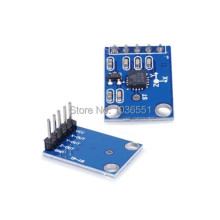# How to use the ADXL355 or GY-61 accelrometer

Though I used the GY-61, the ADXL355Attach the pins to the shown on arduino. The code is attached. just open the serial monitor, upload the program and you're set!!
Im 11 years old, so be nice.``````/*
note:vcc-->5v ,but ADXL335 Vs is 3.3V
The circuit:
5V: VCC
analog 1: x-axis
analog 2: y-axis
analog 3: z-axis
*/
const int xpin = 1;                  // x-axis of the accelerometer
const int ypin = 2;                  // y-axis
const int zpin = 3;                  // z-axis (only on 3-axis models)
void setup()
{
// initialize the serial communications:
Serial.begin(9600);
}
void loop()
{

float zero_G = 512.0; //ADC is 0~1023  the zero g output equal to Vs/2
//ADXL335 power supply by Vs 3.3V
float scale = 102.3;  //ADXL335330 Sensitivity is 330mv/g
//330 * 1024/3.3/1000

/*Serial.print(x);
Serial.print("\t");
Serial.print(y);
Serial.print("\t");
Serial.print(z);
Serial.print("\n");*/
Serial.print(((float)x - 331.5)/65*9.8);  //print x value on serial monitor
Serial.print("\t");
Serial.print(((float)y - 329.5)/68.5*9.8);  //print y value on serial monitor
Serial.print("\t");
Serial.print(((float)z - 340)/68*9.8);  //print z value on serial monitor
Serial.print("\n");
delay(500);
}
``````

OH yeah GO India

1 Like

what u want help or sharing code?

Share code yaar

It is true that you describe, 5v into GY-61 (adxl335 + 3v3 LDO 662k on PCB).
LDO looks like a SMD transistor, but is lablet with the text "662K"
But lacking a 3v3 output from LDO to Aref, for more accurate readout.

I think that there must be other than me with this "problem", it can be solved easily, by soldering a wire on the output of the LDO 3v3 pin, but LDO 3v3 pin is small.
And there must be another solution, without buying another module.

If someone has an idea, hardware or software, all can be used.

Hey Zbot,

Thank you so much for sharing this code.

Very helpful for current project. Before using this code according to my needs, can you please explain certain values, that you used.

float zero_G = 512.0; //ADC is 0~1023 the zero g output equal to Vs/2
//ADXL335 power supply by Vs 3.3V

Why you use 512 here? and

float scale = 102.3; //ADXL335330 Sensitivity is 330mv/g
//330 * 1024/3.3/1000

How you got this 102.3 value here?

And what is this calculation for : Serial.print(((float)x - 331.5)/65*9.8);

kunalkalra2001:
float zero_G = 512.0; //ADC is 0~1023 the zero g output equal to Vs/2
//ADXL335 power supply by Vs 3.3V

Why you use 512 here? and

float scale = 102.3; //ADXL335330 Sensitivity is 330mv/g
//330 * 1024/3.3/1000

How you got this 102.3 value here?

I guess the 512 is the requirement for the G-sensor zu be zero_G, since it ist the middle between 0-1023. It might be slightly more effective to set it as an Int since a float takes more space (just a recommendation).

The 102.3 is described in the code (actually 102.4).

I hope I could help

For gyro stabilization.
Is there anyone who built a similar project ?

float zero_G = 512.0; //ADC is 0~1023 the zero g output equal to Vs/2
//ADXL335 power supply by Vs 3.3V

Why you use 512 here? and

float scale = 102.3; //ADXL335330 Sensitivity is 330mv/g
//330 * 1024/3.3/1000

How you got this 102.3 value here?